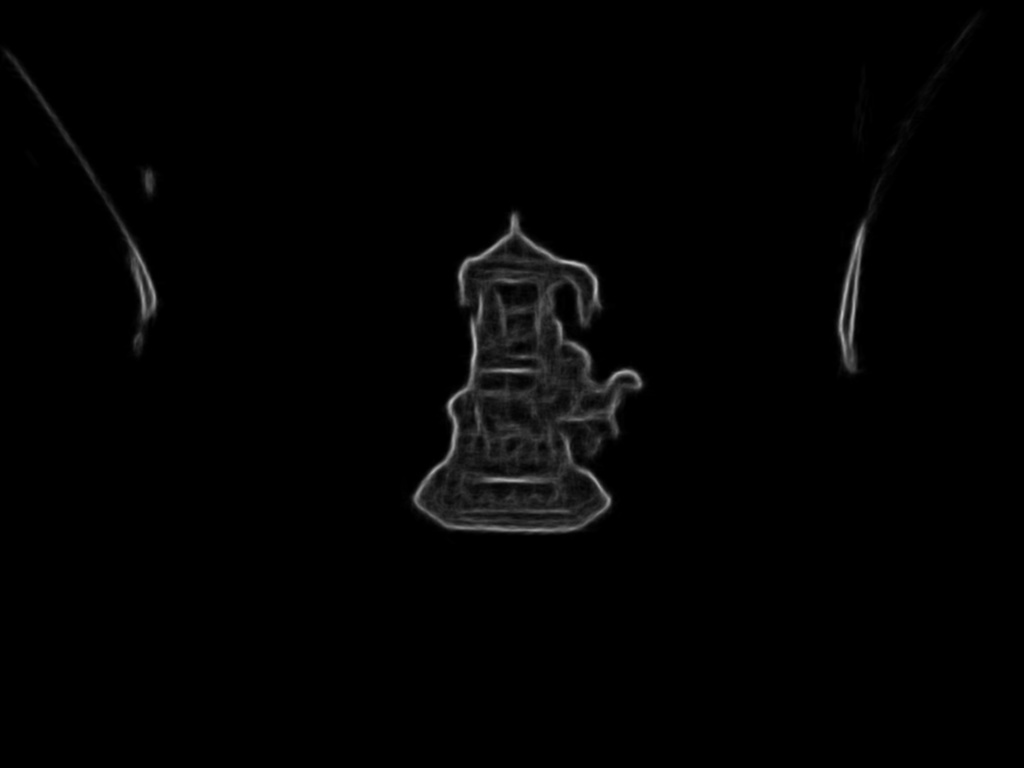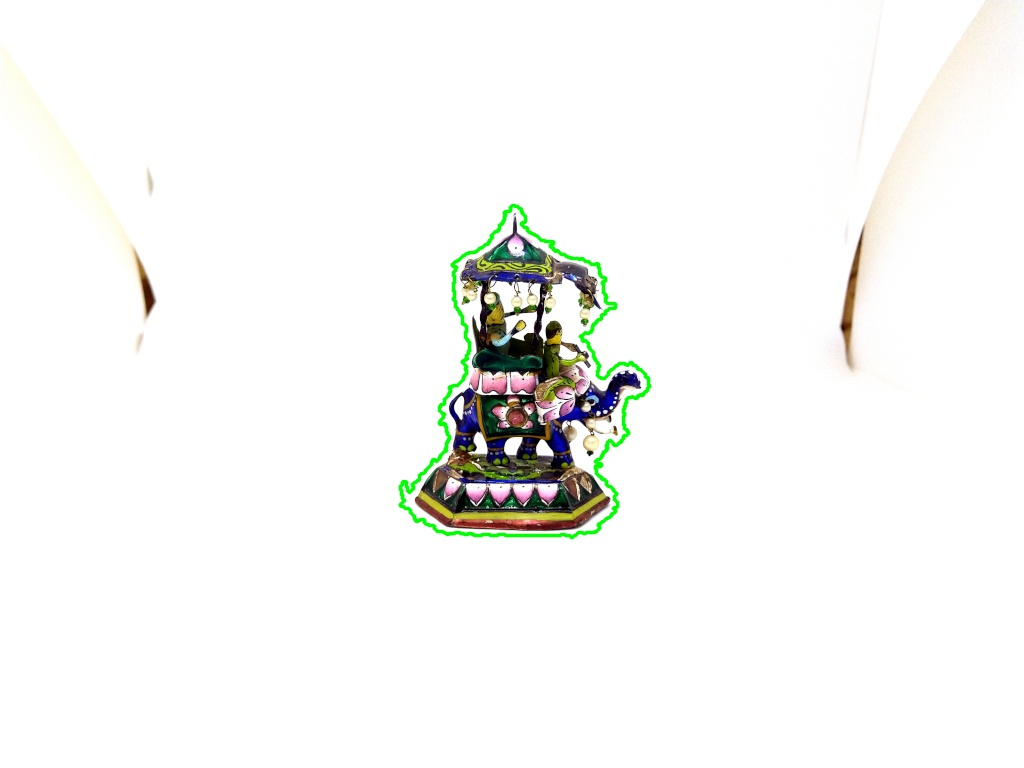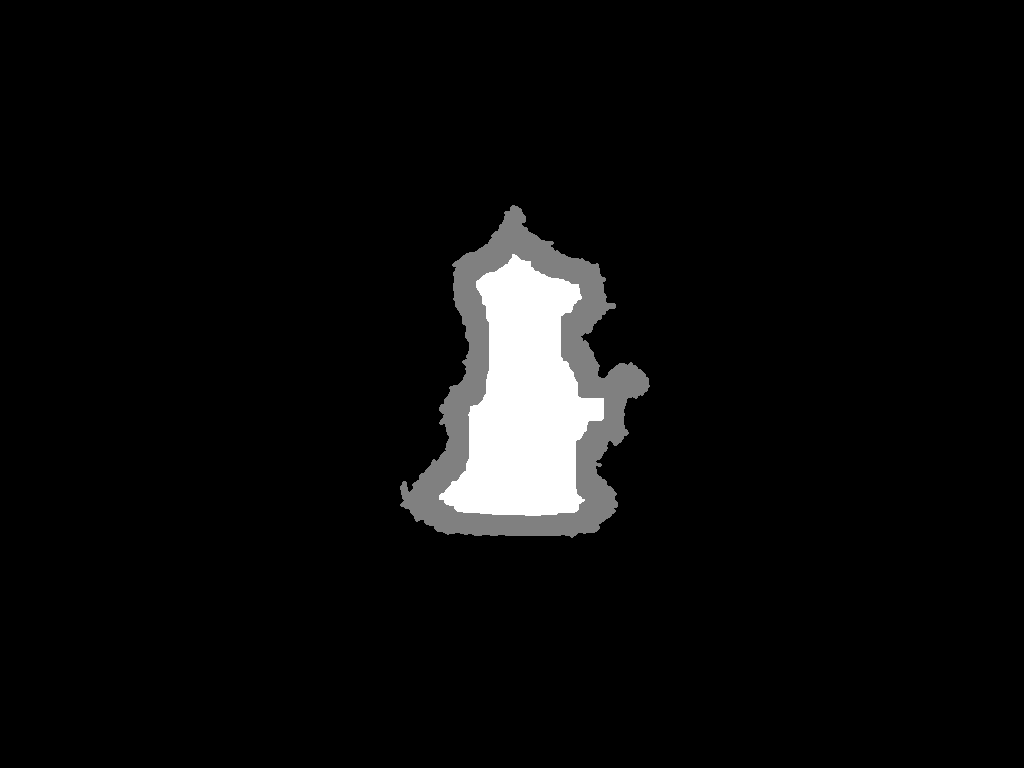Since I last wrote my post on background removal in 2016, I’ve searched for alternative ways to get better results. Here I will dive into my new approach.

At a high level the steps are as follows:

1. Edge detection: Unlike the last time where I used Sobel gradient edges, this time I’ll be using a structured forest ML model to do edge detection
2. Get an approximate contour of the object
3. Use OpenCV’s GrabCut algorithm and the approximate contour to make a more accurate background and foreground differentiation

We are going to use OpenCV 4. You need to also install opencv contrib modules.

``````pip install --user opencv-python==4.5.1.48 opencv-contrib-python==4.5.1.48
``````

With that done, let’s get started. First some gaussian blur to reduce noise.

``````import numpy as np
import cv2

blurred = cv2.GaussianBlur(src, (5, 5), 0)
``````Without this step, there is sometimes too much noise in the edge detection phase.

Next, I do edge detection using a structured forest ML approach from an opencv contribution module. I am not fully aware of the underlying ML technique used by the module. However I can speak from testing several images, that the model does pretty well on edge detection without keeping much noise.

``````blurred_float = blurred.astype(np.float32) / 255.0
edgeDetector = cv2.ximgproc.createStructuredEdgeDetection("model.yml")
edges = edgeDetector.detectEdges(blurred_float) * 255.0
cv2.imwrite('edge-raw.jpg', edges)
``````Next, I filter out further noise using median filters (it is “salt and pepper noise” this time).

``````def filterOutSaltPepperNoise(edgeImg):
# Get rid of salt & pepper noise.
count = 0
lastMedian = edgeImg
median = cv2.medianBlur(edgeImg, 3)
while not np.array_equal(lastMedian, median):
# get those pixels that gets zeroed out
zeroed = np.invert(np.logical_and(median, edgeImg))
edgeImg[zeroed] = 0

count = count + 1
if count > 50:
break
lastMedian = median
median = cv2.medianBlur(edgeImg, 3)

edges_8u = np.asarray(edges, np.uint8)
filterOutSaltPepperNoise(edges_8u)
cv2.imwrite('edge.jpg', edges_8u)
``````This step is necessary again, as the next step (contour detection) is sensitive to noise. You can see from the image that at this point the most prominent edges remain.

Next step: contour detection.

``````def findLargestContour(edgeImg):
contours, hierarchy = cv2.findContours(
edgeImg,
cv2.RETR_EXTERNAL,
cv2.CHAIN_APPROX_SIMPLE
)
``````

Now we need to find the largest contour by area.

``````
# From among them, find the contours with large surface area.
contoursWithArea = []
for contour in contours:
area = cv2.contourArea(contour)
contoursWithArea.append([contour, area])

contoursWithArea.sort(key=lambda tupl: tupl, reverse=True)
largestContour = contoursWithArea
return largestContour

contour = findLargestContour(edges_8u)
# Draw the contour on the original image
contourImg = np.copy(src)
cv2.drawContours(contourImg, [contour], 0, (0, 255, 0), 2, cv2.LINE_AA, maxLevel=1)
cv2.imwrite('contour.jpg', contourImg)
``````For the next step, the major idea is to use grabcut algorithm to get the exact edges. However grabcut requires a hint on sure foreground, sure background and probable foregorund areas. Normally this information is provided manually (i.e. a person needs to mark these areas). However we can approximate it automatically by offsetting the contour to a “reasonably safe” margin.

``````mask = np.zeros_like(edges_8u)

# calculate sure foreground area by dilating the mask
mapFg = cv2.erode(mask, np.ones((5, 5), np.uint8), iterations=10)

# mark inital mask as "probably background"
# and mapFg as sure foreground
trimap[mapFg == 255] = cv2.GC_FGD

# visualize trimap
trimap_print = np.copy(trimap)
trimap_print[trimap_print == cv2.GC_PR_BGD] = 128
trimap_print[trimap_print == cv2.GC_FGD] = 255
cv2.imwrite('trimap.png', trimap_print)
``````Now run grabcut algorithm:

``````# run grabcut
bgdModel = np.zeros((1, 65), np.float64)
fgdModel = np.zeros((1, 65), np.float64)
cv2.grabCut(src, trimap, rect, bgdModel, fgdModel, 5, cv2.GC_INIT_WITH_MASK)

(trimap == cv2.GC_FGD) | (trimap == cv2.GC_PR_FGD),
255,
0
).astype('uint8')
``````You will notice that there might be a problem with the mask. That is, grabcut might leave out some inner parts of the object you are trying to cut, even though the object doesn’t have holes or translucent parts to it. If you are sure that the object is a single polygon, then we can retcify the defect by running contour detection again and filling in the holes.

``````contour2 = findLargestContour(mask2)
``````

Finally you’ve got the pixels that are part of the object. If you just throw away the other pixels now, you will see rough edges around the object. The trick here is to do a blurred blend of the edges to make it look smoother. You may also run a slight contour smoothing algorithm like the Savitzky-Golay filter I mentioned in my last post.

``````# blended alpha cut-out
alpha = mask4.astype(float) * 1.1  # making blend stronger
alpha[alpha > 255] = 255.0

foreground = np.copy(src).astype(float)
background = np.ones_like(foreground, dtype=float) * 255.0

cv2.imwrite('foreground.png', foreground)
cv2.imwrite('background.png', background)
cv2.imwrite('alpha.png', alpha)

# Normalize the alpha mask to keep intensity between 0 and 1
alpha = alpha / 255.0
# Multiply the foreground with the alpha matte
foreground = cv2.multiply(alpha, foreground)
# Multiply the background with ( 1 - alpha )
background = cv2.multiply(1.0 - alpha, background)

cv2.imwrite('cutout.jpg', cutout)
``````The final result looks amazing. Doesn’t it?

Note that I took the initial photo inside a well lit photo box with my phone camera. I then used GIMP to do a white balancing + increasing the exposure (these steps probably can be automated using OpenCV as well).

The photography makes a difference in the edge detection phase. Sharp dark shadows bring unnecessary edges. So it is better to use soft light (softbox or diffusers).

Too much lighting or placing a white product on a white background causes important edges to be too thin. Always place product in a contrasting backdrop. Even in the photo sample above, you will see some white parts from the object were cut-out. I could have avoided it by using a maroon backdrop or something.

You can find full code here. That’s all folks. Hope you enjoyed playing around with this approach.

Update Feb, 2021: Updated post for OpenCV 4, and simplified some code as well